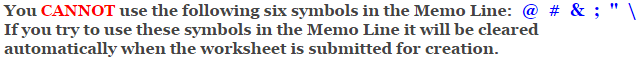# Algebra 2 - Exponential and Logarithmic Functions

## Graphing Exponential Functions Worksheets

This Algebra 2 Graphing Exponential Functions worksheet will give you logarithmic functions to graph. You may choose to graph an equation or write an equation from a graph.

### Language for the Exponential and Logarithmic Worksheet

 English German Albanian Spanish Swedish Italian French Turkish Polish Norwegian

### Memo Line for the Exponential and Logarithmic Worksheet

You may enter a message or special instruction that will appear on the bottom left corner of the Exponential and Logarithmic Worksheet.### Exponential and Logarithmic Worksheet Answer Page

Now you are ready to create your Exponential and Logarithmic Worksheet by pressing the Create Button.

 Recommended Videos

If You Experience Display Problems with Your Math WorksheetMath-Aids.Com.  All rights reserved. Algebra 2 - Exponential Functions Worksheets